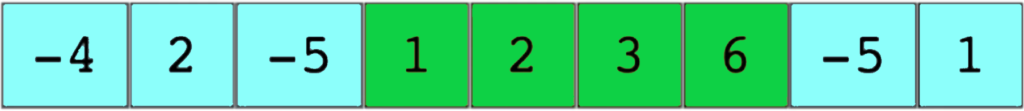In this article, we will understand the idea of Kadane’s Algorithm. We discuss this with the help of an example and also discuss a famous interview problem related to it. Then, we will look at the implementation and analyze the complexities of our approach.

This algorithm is useful in solving the famous ‘Maximum Sum Subarray’ problem. The problem states that given an array we need to find the contiguous subarray with maximum sum and print the maximum sum value. So, how does Kadane’s Algorithm help us in this problem?

The basic idea is to find all contiguous segments of an array whose sum will give us the maximum value. Kadane’s algorithm scans the given array from left to right. In the ith step, it computes the subarray with the largest sum ending at i starting for each subarray.

For example, let us consider this array:For the given array the maximum subarray exists for [ 1 2 3 6] highlighted in the image and the maximum sum is 12.

### Algorithm

Now we look at the algorithm to find the maximum sum subarray.

1. We have two variables max_till_here and max_sum and initialize each variable with the first element of our array.

2. The max_till_here will hold the maximum of all elements or the maximum sum of all elements whichever is greater for a subarray. The max_sum will be our result variable which will contain the maximum of all max_till_here values.

3. So, we start iterating from index 1 or the second element of our array and keep doing the above steps. We keep adding the current array element if its sum with max_till_here is greater than the current element otherwise the max_till_here holds the value of the current element. We also update the max_sum variable with the maximum of max_sum and max_till here on each iteration.

Let us understand these steps with the above mentioned example:

```Given Array arr : [ -4, 2, -5, 1, 2, 3, 6, -5, 1]

Initialize
max_till_here = arr or -4
max_sum = arr or -4

For each iteration, we calculate max_till_here and max_sum as
max_till_here = max(arr[i], max_till_here+arr[i])
max_sum = max(max_sum, max_till_here)

We start at i=1, arr = 2
max_till_here = max(2,-4+2) = max(2,-2) = 2
max_sum = max(-4,2) = 2

At i=2, arr = -5
max_till_here = max(-5,2+(-5)) = max(-5,-3) = -3
max_sum = max(2,-3) = 2

At i=3, arr = 1
max_till_here = max(1,-3+1) = max(1,-2) = 1
max_sum = max(2,1) = 2

At i=4, arr = 2
max_till_here = max(2,1+2) = max(1,3) = 3
max_sum = max(2,3) = 3

At i=5, arr = 3
max_till_here = max(3,3+3) = max(3,6) = 6
max_sum = max(3,6) = 6

At i=6, arr = 6
max_till_here = max(6,6+6) = max(6,12) = 12
max_sum = max(6,12) = 12

At i=7, arr = -5
max_till_here = max(-5,12+(-5)) = max(-5,7) = 7
max_sum = max(12,7) = 12

At i=8, arr = 1
max_till_here = max(1,7+1) = max(1,8) = 8
max_sum = max(12,8) = 12```

This is the working of the above algorithm for the above mentioned example in the image. You can see the max_sum obtained is 12.

### Implementation in Java

Now we look at the implementation of the above discussed example in Java:

```public class KadaneMaximumSumSubarray

static int maximumSubArraySum(int arr[])       //declaring method static help us to call method directly

int n=arr.length;
int max_till_here = arr;                     //Initialize max_till_here and max_sum with
int max_sum = arr;                           // first element of array.

for (int i = 1; i < n; i++)                     // We start iterating from second element.

max_till_here = Math.max(arr[i], max_till_here + arr[i]);
max_sum = Math.max(max_sum, max_till_here);

return max_sum;                             // At the end return max_sum which contain maximumSubArraySu

/* Driver Code to test above methods */
public static void main(String[] args)

int arr[] = -4, 2, -5, 1, 2, 3, 6, -5, 1;
int max_sum = maximumSubArraySum(arr);             // we call the function to get the result

System.out.println("Maximum Sum of Contiguous Subarray is : "+ max_sum);

```

Output:

`Maximum Sum of Contiguous Subarray is : 12`

Note: The above discussed approach also handles the case when the input array has all elements negative. In that case, the maximum element of array is our output.

Now, let us have a look at the time and space complexities of Kadane’s algorithm implementation in calculating the maximum subarray sum.

Time Complexity: We traverse the whole array only once while performing operations that require constant time so the time complexity is O(n).

Space Complexity: We do not use any auxiliary space so complexity is O(1).

That’s all for the article the algorithm is explained above you can try it out with other examples with the code.

Let us know if you have any queries in the comment section below.

The post Kadane’s Algorithm (Maximum Sum Subarray Problem) in Java appeared first on The Crazy Programmer.